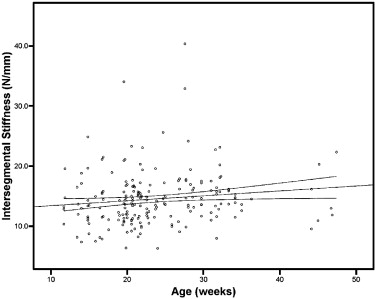INTRODUCING THE EXTERNAL LINK MODEL FIGURE 7Fig 7

Age effect on spine stiffness.

This scatterplot examines spine stiffness change with age for all study animals without links. The regression line (solid dark line) shows that increasing age is not associated with a significant increase in spine stiffness over the range of ages in this study, 13 to 48 weeks. The diverging lines represent the 95% confidence interval around the regression line. Spinal attachment unit–bearing control animals (CSAU) and experimental animals (EN, EF, and ER), before linking, were pooled for the analysis. The regression line is described by the equation Y = 12.60 + 0.08X. Only approximately 2% of intersegmental stiffness can be explained by age (r2 = 0.02).